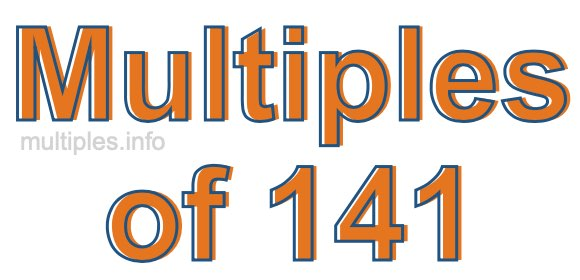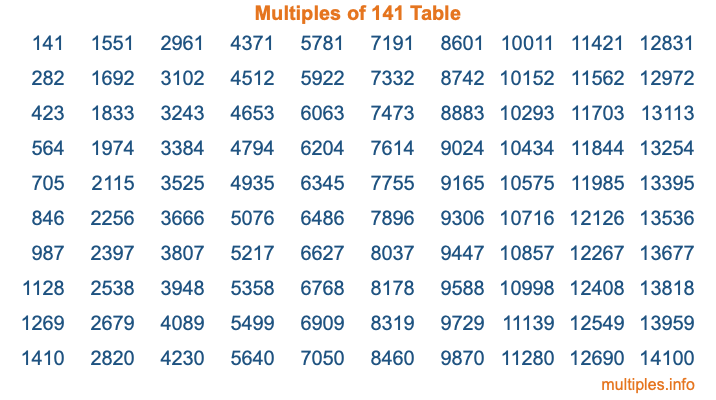Multiples of 141Welcome to the Multiples of 141 page. Here we will first teach you everything you will ever need to know about the multiples of 141, and then give you a study guide summary of everything we taught you to make sure you remember it all. Use this page to look up facts and learn information about the multiples of 141. This page will make you a multiples of one hundred forty-one expert!

Definition of Multiples of 141
Multiples of 141 are all the numbers that when divided by 141 equal an integer. Each of the multiples of 141 are called a multiple. A multiple of 141 is created by multiplying 141 by an integer.

Therefore, to create a list of multiples of 141, you start with 1 multiplied by 141, then 2 multiplied by 141, then 3 multiplied by 141, and so on for as long as you want. Thus, the list of the first five multiples of 141 is 141, 282, 423, 564, and 705. To see a larger list of multiples of 141, see the printable image of Multiples of 141 further down on this page. We also have a category where you can choose any nth multiple of 141.

Multiples of 141 Checker
The Multiples of 141 Checker below checks to see if any number of your choice is a multiple of 141. In other words, it checks to see if there is any number (integer) that when multiplied by 141 will equal your number. To do that, we divide your number by 141. If the the quotient is an integer, then your number is a multiple of 141.

Is  a multiple of 141?

Least Common Multiple of 141 and ...
A Least Common Multiple (LCM) is the lowest multiple that two or more numbers have in common. This is also called the smallest common multiple or lowest common multiple and is useful to know when you are adding our subtracting fractions. Enter one or more numbers below (141 is already entered) to find the LCM.

Check out our LCM Calculator if you need more details about the Least Common Multiple or if you need the LCM for different numbers for adding and subtraction fractions.

nth Multiple of 141
As we stated above, 141 is the first multiple of 141, 282 is the second multiple of 141, 423 is the third multiple of 141, and so on. Enter a number below to find the nth multiple of 141.

th multiple of 141

Multiples of 141 vs Factors of 141
141 is a multiple of 141 and a factor of 141, but that is where the similarities end. All postive multiples of 141 are 141 or greater than 141. All positive factors of 141 are 141 or less than 141.

Below is the beginning list of multiples of 141 and the factors of 141 so you can compare:

Multiples of 141: 141, 282, 423, 564, 705, etc.

Factors of 141: 1, 3, 47, 141

As you can see, the multiples of 141 are all the numbers that you can divide by 141 to get a whole number. The factors of 141, on the other hand, are all the whole numbers that you can multiply by another whole number to get 141.

It's also interesting to note that if a number (x) is a factor of 141, then 141 will also be a multiple of that number (x).

Multiples of 141 vs Divisors of 141
The divisors of 141 are all the integers that 141 can be divided by evenly. Below is a list of the divisors of 141.

Divisors of 141: 1, 3, 47, 141

The interesting thing to note here is that if you take any multiple of 141 and divide it by a divisor of 141, you will see that the quotient is an integer.

Multiples of 141 Table
Below is an image of the first 100 multiples of 141 in a table. The table is in chronological order, column by column. The first column has the first ten multiples of 141, the second column has the next ten multiples of 141, and so on.The Multiples of 141 Table is also referred to as the 141 Times Table or Times Table of 141. You are welcome to print out our table for your studies.

Negative Multiples of 141
Although not often discussed or needed in math, it is worth mentioning that you can make a list of negative multiples of 141 by multiplying 141 by -1, then by -2, then by -3, and so on, to get the following list of negative multiples of 141:

-141, -282, -423, -564, -705, etc.

Multiples of 141 Summary
Below is a summary of important Multiples of 141 facts that we have discussed on this page. To retain the knowledge on this page, we recommend that you read through the summary and explain to yourself or a study partner why they hold true.

There are an infinite number of multiples of 141.

A multiple of 141 divided by 141 will equal a whole number.

141 divided by a factor of 141 equals a divisor of 141.

The nth multiple of 141 is n times 141.

The largest factor of 141 is equal to the first positive multiple of 141.

141 is a multiple of every factor of 141.

141 is a multiple of 141.

A multiple of 141 divided by a divisor of 141 equals an integer.

141 divided by a divisor of 141 equals a factor of 141.

Any integer times 141 will equal a multiple of 141.

Multiples of a Number
Here you can get the multiples of another number, all with the same attention to detail as we did for multiples of 141 on this page.

Multiples of
Multiples of 142
Did you find our page about multiples of one hundred forty-one educational? Do you want more knowledge? Check out the multiples of the next number on our list!